Courses

# Test: Past 28 Years Questions: Semiconductor Electronics- 3

## 25 Questions MCQ Test Physics 28 Years Past year papers for NEET/AIPMT Class 12 | Test: Past 28 Years Questions: Semiconductor Electronics- 3

Description
This mock test of Test: Past 28 Years Questions: Semiconductor Electronics- 3 for NEET helps you for every NEET entrance exam. This contains 25 Multiple Choice Questions for NEET Test: Past 28 Years Questions: Semiconductor Electronics- 3 (mcq) to study with solutions a complete question bank. The solved questions answers in this Test: Past 28 Years Questions: Semiconductor Electronics- 3 quiz give you a good mix of easy questions and tough questions. NEET students definitely take this Test: Past 28 Years Questions: Semiconductor Electronics- 3 exercise for a better result in the exam. You can find other Test: Past 28 Years Questions: Semiconductor Electronics- 3 extra questions, long questions & short questions for NEET on EduRev as well by searching above.
QUESTION: 1

### The following truth table belongs to which of the following four gates?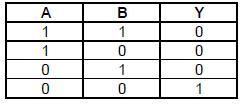Solution:

The given truth table is of
(OR gate + NOT gate) ≡ NOR gate

QUESTION: 2

### A semi-conducting device is connected in aseries circuit with a battery and a resistance. Acurrent is found to pass through the circuit. Ifthe polarity of the battery is reversed, the currentdrops to almost zero. The device may be

Solution:

In reverse bias, the current through a p-n
junction is almost zero.

QUESTION: 3

### The transfer ratio β of a transistor is 50. Theinput resistance of the transistor when used inthe common emitter configuration is 1 kΩ. Thepeak value of the collector A.C. current for anA.C. input voltage of 0.01 V peak is 

Solution: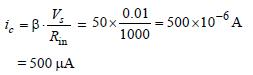QUESTION: 4

Which of the following gates will have an output of 1?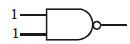(a)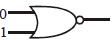(b)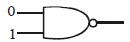(c)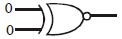(d)

Solution:

(a) is a NAND gate so output is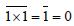(b) is a NOR gate so output is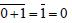(c) is a NAND gate so output is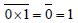(d) is a XOR gate so output is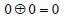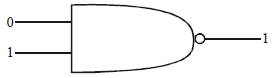Following is NAND Gate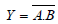QUESTION: 5

The truth-table given below is for which gate?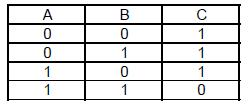Solution:

Relation between A, B and C shows that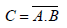QUESTION: 6

The cause of the potential barrier in a p-n diodeis 

Solution:

During the formation of a junction diode,
holes from p-region diffuse into n-region and
electrons from n-region diffuse into p-region.
In both cases, when an electron meets a hole,
they cancel the effect of each other and as a
result, a thin layer at the junction becomes
free from any of charge carriers. This is called
depletion layer. There is a potential gradient
in the depletion layer, negative on the p-side
and positive on the n-side. The potential
difference thus developed across the
junction is called potential barrier.

QUESTION: 7

In forward bias, the width of potential barrier ina p-n junction diode 

Solution:

We know that in forward bias of p-n junction
diode, when positive terminal is connected
to p-type diode, the repulsion of holes takes
place which decreases the width of potential
barrier by striking the combination of holes
and electrons.

QUESTION: 8

A depletion layer consists of 

Solution:

Depletion layer is formed by diffusion of
holes and electrons from p-type
semiconductor to n-type semiconductor and
vice-versa. Hence, donor and acceptor atom
get positive and negative charge leading to
formation of p-n junction. Thus, donor and
acceptor are immobile.

QUESTION: 9

Which of the following when added acts as animpurity into silicon produced n-type semiconductor?    

Solution:

n-type of silicon semiconductor is formed
when impurity is mixed with pentavalent
atom. Out of given choices only phosphorus
is pentavalent.

QUESTION: 10

In a junction diode, the holes are due to        

Solution:

Holes are produced due to missing of
electrons.

QUESTION: 11

A gate has the following truth table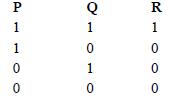The gate is

Solution:

P, Q and R are related as R = P. Q which is
relation of AND gate.

QUESTION: 12

In the study of transistor as amplifier, if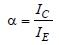and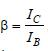, where, IC, IB and IE are the collector, base and emitter currents, then   

Solution:

As we know that Ie = Ic = Ib
Divide both side by Ie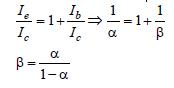QUESTION: 13

The forward biased diode is            

Solution: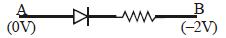As A is at higher potential than B, hence
current will flow from A to B i.e. from p
junction to n junction and diode is forward
biased.

QUESTION: 14

Which gate is represented by the following truth table ?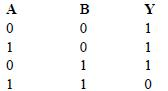Solution: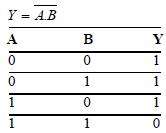Which is truth table of NAND gate

QUESTION: 15

The intrinsic semiconductor becomes insulator at



Solution:

At 0K, motion of free electrons stop. Hence
conductivity becomes zero. Therefore, at 0K
intrinsic semiconductor becomes insulator.

QUESTION: 16

For a common emitter circuit if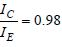then  current gain for common emitter circuit will be   

Solution:

We have,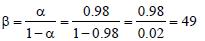QUESTION: 17

Transmission of light in optical fibre is due to         

Solution:

Light is trasmitted through optical fibre due
to multiple total internal reflections.

QUESTION: 18

A d.c. battery of V volt is connected to a series combination of a resistor R and an ideal diode D as shown in the figure below. The potential difference across R will be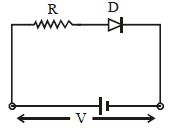Solution:

In forward biasing, the diode conducts. For
ideal junction diode, the forward resistance
is zero; therefore, entire applied voltage
occurs across external resistance R i.e., there
occurs no potential drop, so potential across
R is V in forward biased.

QUESTION: 19

In a p-n junction     

Solution:

For conduction, p-n junction must be
forward biased. For this p-side should be
connected to higher potential and n-side to
lower potential.

QUESTION: 20

In the case of a common emitter transistoramplifier, the ratio of the collector current to theemitter current Ic /Ie is 0.96. The current gain ofthe amplifier is 

Solution: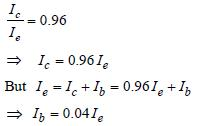QUESTION: 21

If a full wave rectifier circuit is operating from50Hz mains, the fundamental frequency in theripple will be     

Solution:

In case of full wave rectifier,
Fundamental frequency = 2 × mains
frequency
= 2 × 50 = 100Hz.

QUESTION: 22

An n-p-n transistor conducts when 

Solution:

When the collector is positive and emitter is
negative w.r.t. base, it causes the forward
biasing for each junction, which causes
conduction of current.

QUESTION: 23

Barrier potential of a p-n junction diode doesnot depend on 

Solution:

Barrier potential does not depend on diode
design while barrier potential depends upon
temperature, doping density, and forward
biasing.

QUESTION: 24

Following diagram performs the logic function of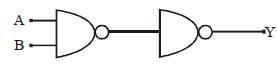Solution: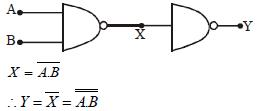Y = A. B by Demorgan therorem
∴ This diagram performs the function of
AND gate.

QUESTION: 25

The following circuit represents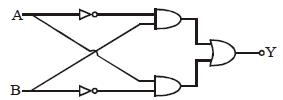Solution:

Output of upper AND gate =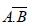Output of lower AND gate =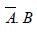∴ Output of OR gate, Y =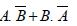This is Boolean expression for XOR gate.